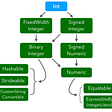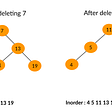# Leetcode — 202. Happy Number

The link to the problem: https://leetcode.com/problems/happy-number/

A happy number is a number defined by the following process:

• Starting with any positive integer, replace the number by the sum of the squares of its digits.
• Repeat the process until the number equals 1 (where it will stay), or it loops endlessly in a cycle which does not include 1.
• Those numbers for which this process ends in 1 are happy.

Return `true` if `n` is a happy number, and `false` if not.

`Example Input: n = 19Output: trueExplanation:1² + 9² = 828² + 2² = 686² + 8² = 1001² + 0² + 0² `

# Thoughts:

From reading the definition of the happy number, it is kind of straightforward to write the code of calculating the sum of the squares of the given number’s digits. We can just use a loop and use modulo and division to help us get the current last digit (n mod 10) and abandon the last digit (n divided by 10).

For example, if we have a number like 123, then 123 mod 10 will help us get the last digit, and 123 divided by 10 will help us get rid of the last digit.

Hence, we can use the below helper method to find the number that is going to be our new n:

`public int helper(int n){        int sum = 0;        while(n>0)        {            int lastDigit = n%10;            sum += lastDigit*lastDigit;            n/=10; //abandon the last digit        }        return sum;    }`

and we will keep calling this helper method by passing n until n is 1 OR until we found that we are in a cycle.

So the biggest question is, how can we find a cycle if there’s one?

The easiest way to do it is by using a set to store all the numbers we have seen before. Once we found we have passed the number into the helper method before, it means that’s the start of the cycle and we can just return false since we already know using that number won’t help us find 1.

Below is the 1ms code that beats 84% — (the details of the helper method is above)

`public boolean isHappy(int n) {        Set<Integer> set = new HashSet<>();        while(n>0)        {            n = helper(n);            if(n==1)                return true;            if(set.contains(n))                return false;            set.add(n);        }        return false;    }`

If you have practiced 141. Linked List Cycle before, you might find that the idea of this question is actually surprisingly similar! Yes, we can also use “two pointers — slow pointer and fast pointer” to help us find a cycle.

1. slow — represents the result of calling the helper method one time at a time.
2. fast — represents the result of calling the helper method twice at a time.

We will find there’s a cycle if slow and fast get the same result.

Below is the 0ms code that beats 100% — (the details of the helper method is above)

`public boolean isHappy(int n) {        int slow = n, fast = n;        while(slow!=1 || fast!=1)        {            slow = helper(slow);            fast = helper(helper(fast));            if(slow==1 || fast==1)                return true;            if(slow==fast)                return false;        }        return true;    }`

--

--

--

## More from Anj

Click here to view all the stories posted on this blog: https://anj910.medium.com/bookmark-c95e4c52cf44

Love podcasts or audiobooks? Learn on the go with our new app.

## Five9 Integration## Get started with Docker and Docker Compose## Isolation Levels in Relational Databases## Infrastructure as Code (IaC)## Swift’s Numeric Protocol## Kotlin: Aesthetics vs Pragmatism## Achieving Clean Architecture with MVVM on Android## The marketplace is live — learn what to do with your NFTs## Anj

Click here to view all the stories posted on this blog: https://anj910.medium.com/bookmark-c95e4c52cf44

## Deleting a Node from Binary Search Tree## Greedy Algorithms